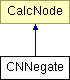# CNNegate Class Reference

Calculation node negating the value of the operand subtree. More...

`#include <expression.h>`

Inheritance diagram for CNNegate:List of all members.

## Public Member Functions

CNNegate (CalcNode *_node)
virtual ~CNNegate ()
virtual double evaluate () const
evaluate the complete calculation tree and return the floating point result value
virtual void print (std::ostream &os, unsigned int depth) const
output the calculation tree to the given stream.

## Private Attributes

CalcNodenode
calculation subtree

## Detailed Description

Calculation node negating the value of the operand subtree.

Definition at line 63 of file expression.h.

## Constructor & Destructor Documentation

 CNNegate::CNNegate ( CalcNode * _node ) ` [inline, explicit]`

Definition at line 69 of file expression.h.

 virtual CNNegate::~CNNegate ( ) ` [inline, virtual]`

Definition at line 74 of file expression.h.

References node.

## Member Function Documentation

 virtual double CNNegate::evaluate ( ) const` [inline, virtual]`

evaluate the complete calculation tree and return the floating point result value

Implements CalcNode.

Definition at line 79 of file expression.h.

References CalcNode::evaluate(), and node.

 virtual void CNNegate::print ( std::ostream & os, unsigned int depth ) const` [inline, virtual]`

output the calculation tree to the given stream.

tries to format the output to make tree levels visible.

Implements CalcNode.

Definition at line 84 of file expression.h.

References CalcNode::indent(), node, and CalcNode::print().

## Member Data Documentation

 CalcNode* CNNegate::node` [private]`

calculation subtree

Definition at line 66 of file expression.h.

Referenced by evaluate(), print(), and ~CNNegate().

The documentation for this class was generated from the following file:

Generated on Sun Aug 3 12:34:09 2008 for Flex Bison C++ Example by1.5.5